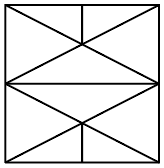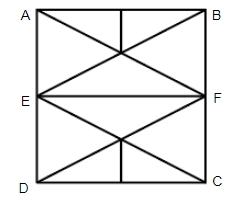Question 3

# How many triangles are present in the given figure?SolutionNumber of triangles in ABFE = 10
Number of triangles in EFCD = 10
And 2 triangles are AFD and CEB.
Total number of triangle = 10 + 10 + 2 = 22
$$\therefore$$ The correct answer is option C.

• Free SSC Study Material - 18000 Questions
• 230+ SSC previous papers with solutions PDF